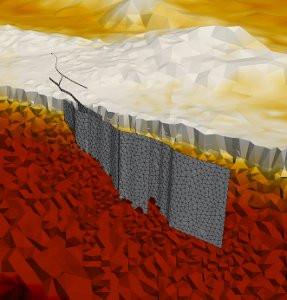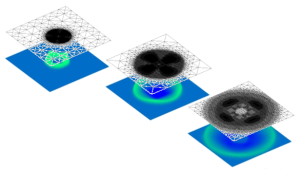### Current Research Topics:

• High performance computing (current application focus is earthquake and tsunami simulation)
• Efficient algorithms and software in computational science and engineering
• Parallel adaptive mesh refinement algorithms for adaptive triangular grids using Sierpinski curves
• Partitioning and load balancing based on space-filling curves
• Hierarchically structured numerical algorithms and their parallelization on modern HPC platforms### Current Projects:

#### Previous Research Topics:

• On the use of block recursive approaches based on Peano's curve to efficiently implement matrix operations
• Development of efficient solvers for partial differential equations using recursive substructuring techniques
• Development of multigrid methods for convection dominated flows
• Using hierarchical bases for the numerical solution of helmholtz equations